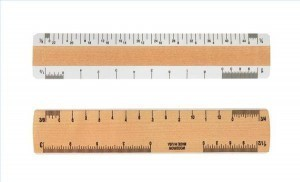•• # Metric System

The following metric system chart shows some metricmeasurements (SI units) and their equivalents with American measurements. Some UK measurements are also included.

### Length Conversions

2.54 centimeters is equal to 1 inch (both in the US and imperial systems). 30.48 centimeters is equivalent to 1 foot (12 inches) while 0.91 meters is equal to 1 yard. 1.61 kilometers is equal to 1 mile.

One centimeter is equal to 0.39 inches and 1 meter is the same as 3.28 feet. 1 meter is equal to 1.09 yards and 1 kilometer is equal to 0.62 miles.

### Weight and Mass Conversion Guide

An ounce is equal to 28.35 grams in the metric system chart. One pound is equal to 0.45 kilograms, while the UK ton (2240 pounds) is equivalent to 1.02 metric tons.

By contrast, the US ton (2000 pounds) is equal to 0.91 metric tons. A gram is equal to 0.035 ounces and a kilogram is equal to 2.21 pounds. The metric ton (1000 kg.) is equal to 0.98 UK tons. The 0.98 UK tons is equivalent to 1.10 US tons.

### Temperature Conversion

Metric measurements use Celsius while those in the US use Fahrenheit. The formulas for converting between the two are C = (F – 32) ÷ 1.8 and F = (C x 1.8) + 32. The freezing points are 0 C and 32 F. The average room temperature is 20 C or 68 F and the normal body temperature is 37 C or 98.6F. The water boiling point is 100 C or 212 F.

Note: officially, the metric system has replaced the Celsius with the Kelvin system of monitoring temperatures. However, the Celsius is still widely used and the Kelvin system has only been employed for scientific purposes and research.

### Area Conversion Guide

0.40 hectare is equal to an acre, while 6.45 square centimeters is equivalent to a square inch. An area of 0.09 square meters is equal to a square foot, while 0.84 square meters is equal to a square yard. 2.60 square kilometers is, in US measurements, equivalent to a square mile.

0.028 cubic meters is the same as a cubic foot and 0.76 cubic meters in the metric system is equal to one cubic yard.One hectare is equal to 2.47 acres, while a square centimeter is equal to 0.16 square inches.

The square meter has as its equivalent 1.20 square yards. A square kilometer is equal to 0.39 square miles while a cubic meter is the same as 35.23 cubic feet. A cubic meter is also equal to 1.35 cubic yards.

### Volume Capacity Conversion Guide

1 US teaspoon is equal to 5.92 milliliters, while a tablespoon is 14.79 milliliters in the SI unit of measurement. One US fluid ounce is equivalent to 29.57 milliliters. A pint is equivalent to 0.47 liters and the US quart is equal to 3.79 liters. A milliliter is equal to 0.20 teaspoons and 10 milliliter is equal to 0.68 tablespoons (US).

This metric system conversion chart lists only some of the most commonly used figures. There are others but they are not as widely employed in common usage.